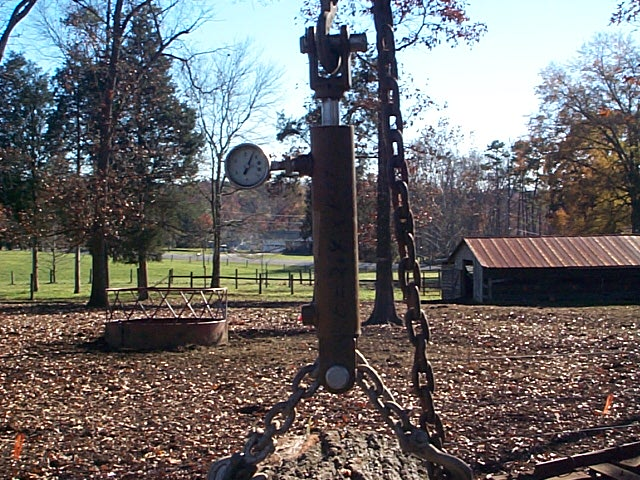#### Hanging Scales using a hydraulic cylinder and pressure gauge

View of 2'' bore and 1" rod by 6'' stroke hydraulic cylinder supporting an eight feet by 40" (on butt end) red oak log. To determine the weight recall that pressure (P) is related to the weight (W) and the difference in the areas (A) of the bore and rod through the formula W = P x 3.14 ( R2 - r2) where R refers to bore radius and r to rod radius. For this example W = P x 3.14( 1 - 1/4) or W = 2.36 x P with P in PSI units.The gauge reads 1400 PSI so the weight of this log is 3300 lbs.